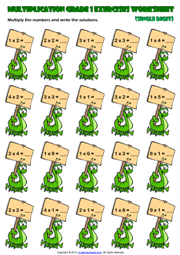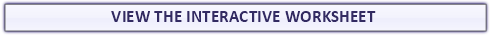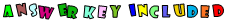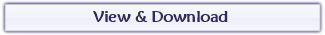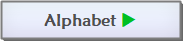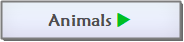Found a mistake?
!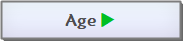## Single Digit Multiplication Maths Exercise WorksheetA fun multiplication exercises maths worksheet for grade 1 (first grade) students and kids with ships and sailor theme. Multiply the numbers and write the solutions. (Vertical Multiplication Worksheet for kids)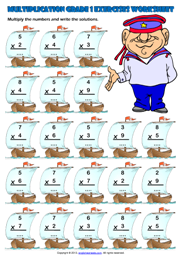## First Grade Single Digit Multiplication Exercise Worksheet

An easy multiplication exercise maths worksheet for grade 1 (first grade) students and kids with caterpillars theme. Multiply the numbers and write the solutions. Single Digit Multiplicants, Multipliers and results.
(Horizontal
Multiplication Worksheet for kids)